[an error occurred while processing this directive]Home > College of Sciences > Institute of Fundamental Sciences > Maths First > Online Maths Help > Calculus > Differentiation > The Sign of the Second Derivative > Concave Up, Concave Down, Points of Inflection SEARCH MASSEY[an error occurred while processing this directive]

# The Sign of the Second Derivative

## Concave Up, Concave Down, Points of Inflection

We have seen previously that the sign of the derivative provides us with information about where a function (and its graph) is increasing, decreasing or stationary. We now look at the "direction of bending" of a graph, i.e. whether the graph is "concave up" or "concave down".

A graph is said to be concave up at a point if the tangent line to the graph at that point lies below the graph in the vicinity of the point and concave down at a point if the tangent line lies above the graph in the vicinity of the point.A point where the concavity changes (from up to down or down to up) is called a point of inflection (POI); note that the tangent line to a graph at a point of inflection must cross the graph at that point.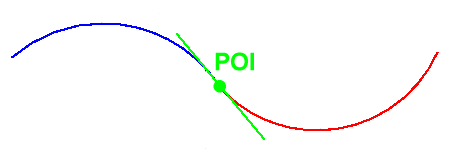Now let's look at concavity from a slightly different perspective. Consider the graph of a cost function shown below. The graph shows that the total cost of a certain activity increases sharply at the beginning and then rises more and more slowly until a point when the total cost begins to rise more sharply again. The blue colour indicates a region where the slope of the tangent decreases. That is, in this region the rate at which the cost function increases, decreases. The red colour indicates a region where the slope of the tangent increases, i.e. the rate at which the cost function increases also increases. By our previous definitions, the blue area is concave downward and the red area is concave upwards. The green point is the point at which the rate of change of the slope changes from decreasing to increasing. It is also the point at which the concavity of the function changes from downward to upward. This point is called a point of inflection (POI).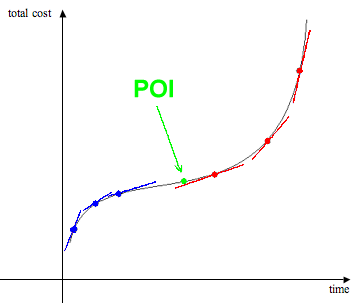In general, note that regardless of the sign of the slope (positive, negative or zero), the slopes of the tangent are decreasing as we move from left to right when the graph is concave down and increasing (from left to right) when it is concave up.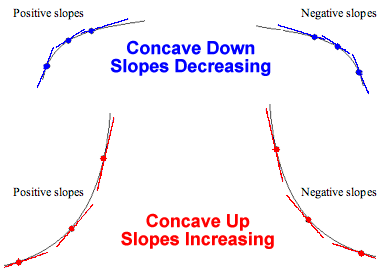### Exercise

Look at the applet below. Note that the function is shown in the top line, the function's graph on the left and it's derivative's graph on the right. Use the slider to change the value of x.  Convince yourself that the graph of the given function f is concave up where the derivative f ' (the slope of the tangent) is an increasing function, and concave down where the derivative f ' is a decreasing function.

Examine the fourteen examples provided in the scroll bar on the top of the applet or enter your own function in the space below the graphs and press new function to display the graphs. To enter your own function, you must use the symbols + for add, for subtract, * for multiply,  / for divide, and ^ to raise to a power. You can also use various mathematical functions: sin, cos, tan, sec, cot, csc, arcsin, arccos, arctan, exp, ln, log2, log10, abs, sqrt and cubert. (Here, "abs" is the absolute value function, "sqrt" is the square root function and "cubert" is the cube root function.)

Continue until you are convinced that, in all cases, the graph of the given function f is concave up where the derivative f ' (the slope of the tangent) is an increasing function, and concave down where the derivative f ' is a decreasing function.

 Make sure you understand the following connections between the two graphs. When the function y = f (x) is concave up, the graph of its derivative y = f '(x) is increasing. When the function y = f (x) is concave down, the graph of its derivative y = f '(x) is decreasing. When the function y = f (x) has a point of inflection (changes from concave up to concave down), the graph of its derivative y = f '(x) has a maximum or minimum (and so changes from increasing to decreasing or decreasing to increasing respectively).

Given the above, we can decide whether a function is increasing or decreasing by looking at the sign of its derivative. To decide whether f ' is increasing or decreasing, we must look at the sign of its derivative (the derivative of f '). This derivative of f ' (i.e. the derivative of the derivative of f) is called the second derivative of f and is denoted by f ''.

In terms of the second derivative, we can summarize our earlier discussion as follows

• The graph of y = f (x) is concave upward on those intervals where y = f "(x) > 0.
• The graph of y = f (x) is concave downward on those intervals where y = f "(x) <  0.
• If the graph of y = f (x) has a point of inflection then y = f "(x)  =  0.

### Exercise

Examine the first example given below. Note that the function is shown on the left, the first derivative in the middle and the second derivative on the left.

Test that the three properties noted just above are true.

Examine the fourteen examples provided in the scroll bar on the top of the applet below or enter your own function in the box provided. If you enter your own function, you must use the symbols + for add, for subtract, * for multiply,  / for divide, and ^ to raise to a power. You can also use various mathematical functions: sin, cos, tan, sec, cot, csc, arcsin, arccos, arctan, exp, ln, log2, log10, abs, sqrt and cubert. (Here, "abs" is the absolute value function, "sqrt" is the square root function and "cubert" is the cube root function.)

 Make sure you understand the following connections between the two graphs. The graph of y = f (x) is concave upward on those intervals where y = f "(x) > 0. The graph of y = f (x) is concave downward on those intervals where y = f "(x) <  0. If the graph of y = f (x) has a point of inflection then y = f "(x)  =  0.

We can calculate the second derivative to determine the concavity of the function's curve at any point.

1. Calculate the second derivative.
2. Substitute the value of x.
3. If f "(x) > 0, the graph is concave upward at that value of x.
4. If f "(x) = 0, the graph may have a point of inflection at that value of x. To check, consider the value of f "(x) at values of x to either side of the point of interest.
5. If f "(x) < 0, the graph is concave downward at that value of x.

### Example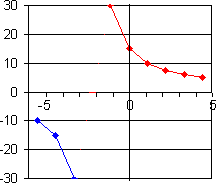What is the shape of the graph of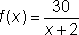at x = 0?

Calculate f "(x).and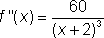When x = 0,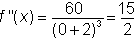f"(x) > 0, so the graph is concave upward at x = 0. In fact f "(x) > 0 for all x > -2 so the graph is concave upward in that range. f "(x) < 0 for all x < -2 so the graph is concave downward if x < -2.

By contrast, consider h(x) = 6, which we know has a horizontal line graph. At all points h '(x) = 0 and h "(x) = 0 but there is no point of inflection.

### Exercise

 What is the shape of the graph of f(x) = x4 + x3 + x2 + x + around x = . Working: concave up concave down point of inflection

Similarly, we can find the points of inflection on a function's graph by calculation.

1. Calculate the second derivative.
2. Solve the equation f "(x) = 0 to obtain the value(s) of x at the possible point(s) of inflection. Check the f "(x) value to either side of each x value to be sure each gives a point of inflection.
3. Substitute the value(s) of x into f(x).
4. Hence deduce the coordinates of the point(s) of inflection.

### Example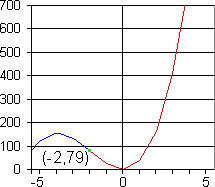If  f(x) = 5x3 + 30x2 + x + 1

then f '(x) = 15x2 + 60x + 1

and f ''(x) = 30x + 60.

At points of inflection, f "(x) = 0. This is true when 0 = 30x + 60, that is x = -2.

f(-2) = 5(-2)3 + 30(-2)2 + (-2) + 1 = 79

Below x = -2, the value of the second derivative, 30x + 60, will be negative so the curve is concave down. For higher values of x, the value of the second derivative, 30x + 60, will be positive so the curve is concave up. We can conclude that the point (-2,79) is a point of inflection.Consider f(x) = x4.

Solving f "(x) = 12x2 = 0 yields x = 0. At values of x < 0, the second derivative is positive. At values of x > 0, the second derivative is positive. (0,0) is a local minimum.

### Exercise

 Calculate the second derivative of: f(x) = x4 + x3 + x2 + x + Hence, calculate the point(s) of inflection. My point(s) of inflection: and